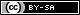# Dev Blog

 ./dev Original theme by orderedlist (CC-BY-SA) Where applicable, all content is licensed under a CC-BY-SA.# Misc. Math

## Landau Notation

$$f(x) = O(g(x)) \iff \limsup_{x \to \infty} \frac{f(x)}{g(x)} < \infty$$

$$f(x) = o(g(x)) \iff \lim_{x \to \infty} \frac{f(x)}{g(x)} = 0$$

$$f(x) = \Omega(g(x)) \iff \liminf_{x \to \infty} \frac{f(x)}{g(x)} > 0$$

$$f(x) = \omega(g(x)) \iff \liminf_{x \to \infty} \frac{f(x)}{g(x)} = \infty$$

$$f(x) = \Theta(g(x)) \iff f(x) = O(g(x)) \ \& \ f(x) = \Omega(g(x))$$

## $e$ is Irrational

Assume $e$ is rational. This means $e = \frac{p}{v}$.

We use the identity:

$$e = \sum_{k=0}^{\infty} \frac{1}{k!}$$

With the usual convention that $0!=1$.

$$\begin{array}{lcl} & e & = \frac{p}{v} \\ & & = \sum_{k=0}^{v} \frac{1}{k!} + \sum_{k=v+1}^{\infty} \frac{1}{k!} \\ \to & (v!) e & = (v-1)! p \\ & & = \sum_{k=0}^{v} \frac{v!}{k!} + ( \frac{1}{v+1} + \frac{1}{(v+1)(v+2)} + \cdots ) \end{array}$$

By assumption, $(v!) e = (v-1)! p \in \mathbb{Z}$. We also have the first sum $\sum_{k=0}^{v} \frac{v!}{k!} \in \mathbb{Z}$.

The second sum is greater than 0 and we can get bounds:

$$\begin{array}{lrcl} & 0 & < \frac{1}{v+1} + \frac{1}{(v+1)(v+2)} + \cdots & \le \frac{1}{v+1} \sum_{k=0}^{\infty} \frac{1}{(v+1)^k} \\ \to & 0 & < \frac{1}{v+1} + \frac{1}{(v+1)(v+2)} + \cdots & \le \frac{1}{v} \end{array}$$

Choose $v > 1$ and we have the second sum as non-integral, contradicting the assumption of rationality.

## Balls and Urns

Balls and urns counting (from src):

Balls Urns unrestricted max(1) min(1)
l l $u^b$ $(u)_b$ $u! { b \brace u }$
u l $\left({u \choose b }\right)$ $u \choose b$ $\left({u \choose b-u }\right)$
l u $\sum_{i=0}^u { b \brace i }$ $[ b \le u ]$ ${ b \brace u }$
u u $\sum_{i=1}^u p_i(b)$ $[ b \le u ]$ $p_u(b)$
• l = labelled
• u = unlabelled
• $(u)_b = \prod_{k=0}^{b-1} (u-k)$
• ${ b \brace u}$ - Stirling's number of the second kind (${ n \brace k } = S(n,k) = k S(n-1,k) + S(n-1,k-1)$)
• $\left({u \choose b }\right) = { n - (k-1) \choose k }$, "with replacement"
• $[ b \le u ]$ = indicator function
• $p_k(n) = p_{k-1}(n-1) + p_k(n-k)$, number of ways to partition $n$ into $k$ parts ($n$ as sum of $k$ integers)

#### ll*

Keep throwing balls without care about occupancy, you get $u^b$.

#### ll?

$u$ choices for the first ball, $(u-1)$ choices for the second ball, etc, until $(u)_b$.

#### ll+

One interpretation of Stirling numbers of the second kind ${ n \brace k } = S(n,k)$ are the way of partitioning $n$ numbers into $k$ subsets.

For example, $S(3,2) = { 3 \brace 2 } = | \{ \{ 1 \} \cup \{2,3\}, \{2\} \cup \{1,3\}, \{3\} \cup \{1,2\} \} |$.

The minimum 1 ball per urn, mans that we have a minimum of $u$ subsets. By definition (?) the urns act as sets themselves, so the order of balls within urns doesn't matter.

We now want to partition $b$ balls into $u$ sets, which is ${ b \brace u }$. Since the urns are labelled, we need to multiply by an extra $u!$ factor, which gives:

$$u! { b \brace u }$$

#### ul*

A stars and bars argument gives the result.

Consider $u$ urns, represented by $u+1$ 'bars' and $b$ balls represented as stars. For example ||***|*|||**|*|...||.

There is a constraint that the ends have bars, so there are $u-1 + b$ symbols in the middle. Choosing all configurations gives ${ u-1+b \choose b }$ configurations, which is $\left({u \choose b }\right)$.

#### ul?

Since balls are unlabeled, we can divide out by the different labelled configurations.

That is, the ll? case divided by $b!$:

$$\frac{(u)_b}{b!} = { u \choose b }$$

#### ul+

This reduces to the unrestricted case (ul*) with first laying down a single ball in each bin.

After the initial outlay, we have $(b-u)$ balls left to put in the ul case, giving $\left({u \choose b-u }\right)$.

#### lu*

One interpretation of Stirling numbers of the second kind ${ n \brace k } = S(n,k)$ are the way of partitioning $n$ numbers into $k$ subsets.

For the unrestricted case, we can think of trying to put $b$ balls into some number of (unlabelled) urns running from $1$ to $u$. That is, for each $i$ from $1$ to $u$, find the number of ways of putting $b$ labels into $i$ subsets:

$$\sum_{i=1}^u { b \brace i }$$

#### lu?

If there are fewer balls than urns, there is only one way to place the balls in unlabeled urns.

#### lu+

One interpretation of Stirling numbers of the second kind ${ n \brace k } = S(n,k)$ are the way of partitioning $n$ numbers into $k$ subsets.

The minimum 1 ball per urn, mans that we have a minimum of $u$ subsets. By definition (?) the urns act as sets themselves, so the order of balls within urns doesn't matter.

We now want to partition $b$ balls into $u$ (unlabelled) sets, which is:

$${ b \brace u }$$

#### uu*

Much like the uu+ case, since the balls and urns are unlabelled, we can think of it as how many ways can the integer $b$ be written as the sum of some number of integers. Since we have no restriction on the minimum number of balls in urns, we have to sum all the different ways to partition, giving:

$$\sum_{i=1}^u p_i(b)$$

#### uu?

If there are fewer balls than urns, there is only one way to place the balls, labelled or no, in unlabeled urns.

#### uu+

Since both balls and urns are unlabelled, this is equivalent to asking how many ways can the integer $b$ be written as the sum of $u$ integers (partitions), which is $p_u(b)$.